# 优秀不够，你是否无可替代

• 随笔 - 382
• 文章 - 0
• 评论 - 271
• 引用 - 0

## 二,ESP8266 GPIO和SPI和定时器和串口(基于Lua脚本语言)

https://www.cnblogs.com/yangfengwu/p/7514336.html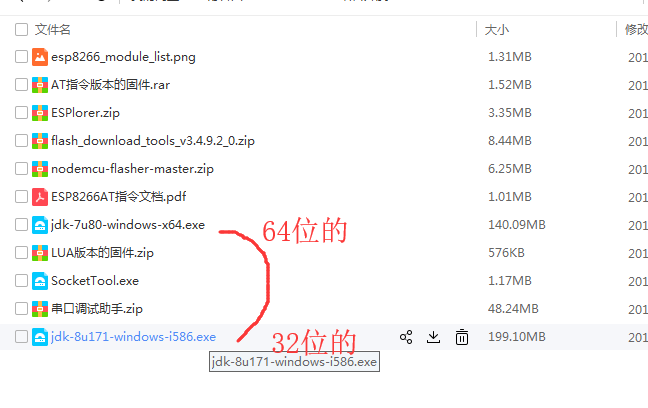http://jingyan.baidu.com/article/3c343ff70bc6ea0d377963df.html让这个灯亮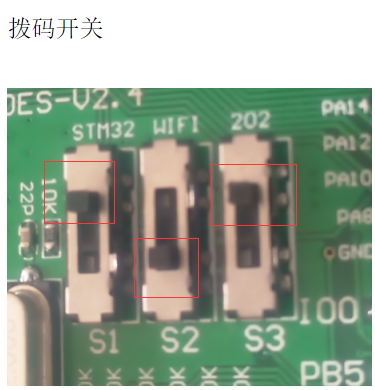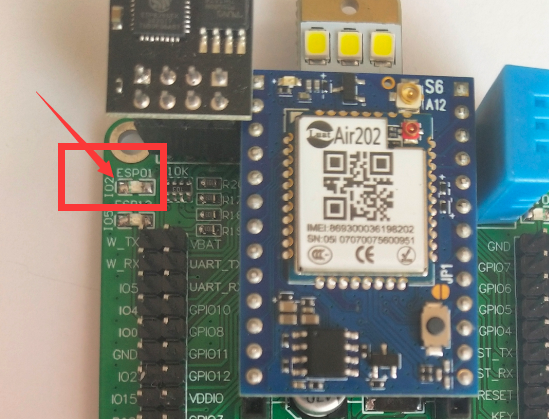gpio.mode(4,gpio.OUTPUT)--输出模式
gpio.write(4,0)--输出低电平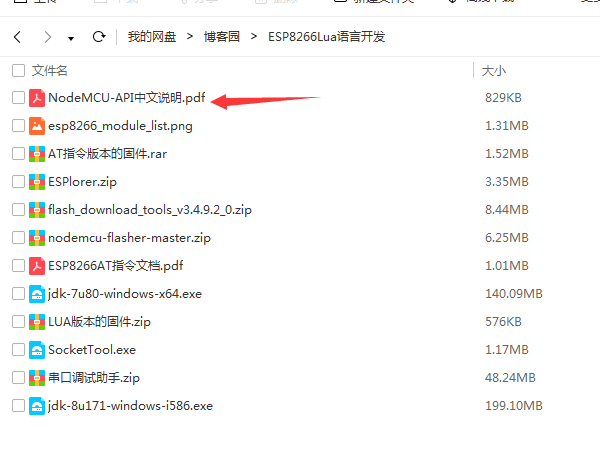关于[html]
1. gpio.mode(4,gpio.OUTPUT)
2. gpio.write(4,1)
3.
4. function aa()--定义一个函数
5.   print("111111")
6. end
7.
8. tmr.alarm(0, 1000, 1, aa)

0代表用的标号为0的定时器

1000代表延时1000ms执行aa

1代表[html]
1. gpio.mode(4,gpio.OUTPUT)
2. gpio.write(4,1)
3.
4.
5. tmr.alarm(1, 1000, 1, function()
6.     print("111111")
7. end)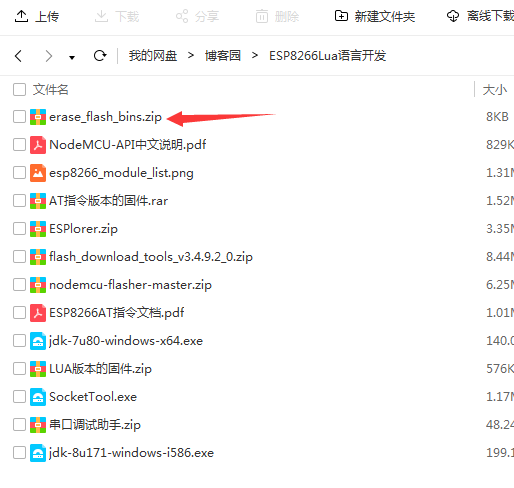http://wiki.ai-thinker.com/_media/esp8266/esp8266_module_list.png等着格式化文件系统,,就等着哈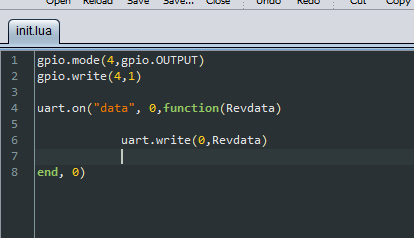gpio.mode(4,gpio.OUTPUT)
gpio.write(4,1)

tmr.alarm(1, 2000, 0, function()

uart.on("data", 0,function(Revdata)

uart.write(0,Revdata)

end, 0)

end)

1.uart.on("data", 0,function(Revdata)
2.
3.            uart.write(0,Revdata)
4.
5.    end, 0)  

uart.write(0,Revdata)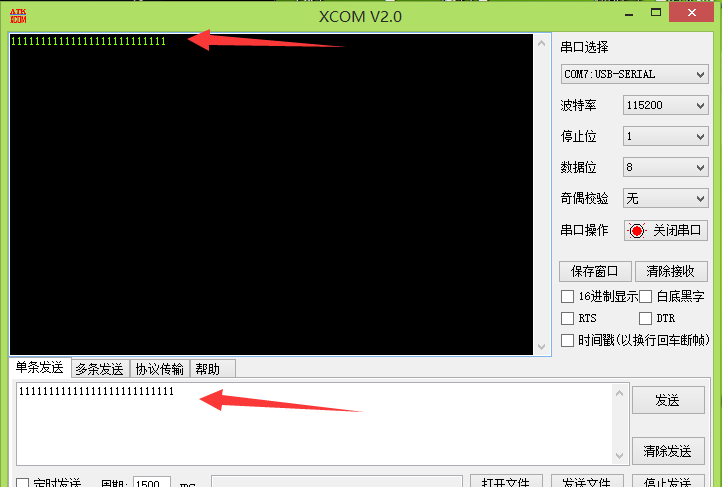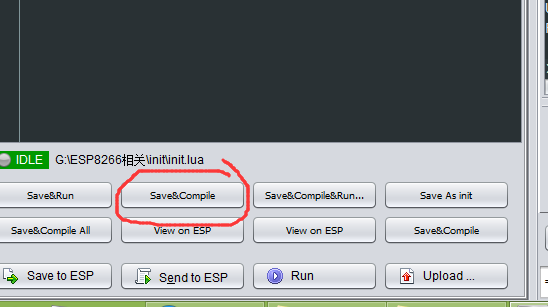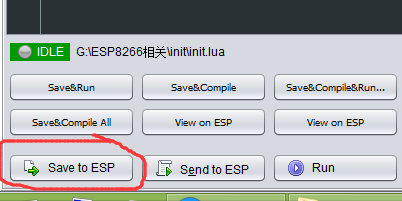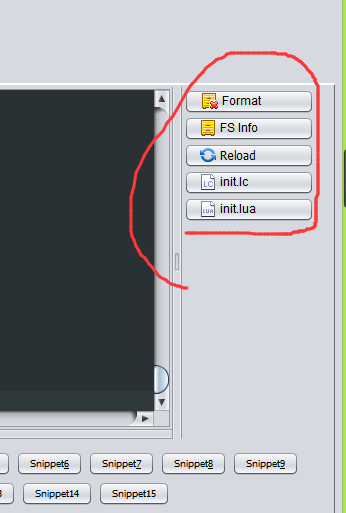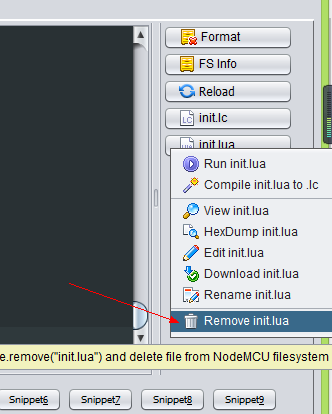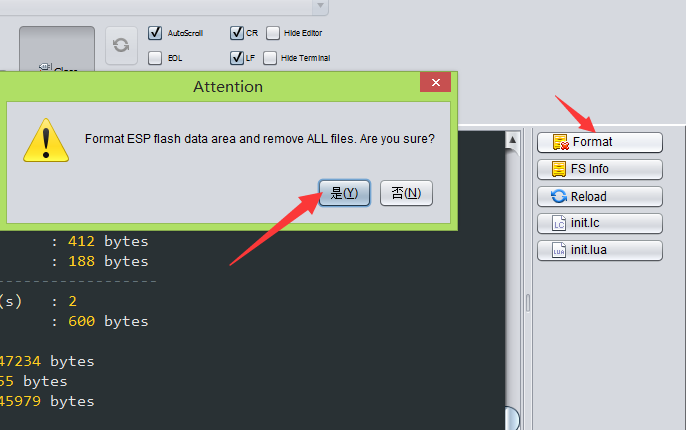print("Relay=1")
print("Relay=0")

[html]

1. gpio.mode(4,gpio.OUTPUT)
2. gpio.write(4,1)
3. tmr.alarm(1, 2000, 0, function()
4.     uart.on("data", 0,function(Revdata)
5.             if Revdata == "H" then
6.                gpio.write(4,1)
7.                print("Relay=1")
8.             end
9.             if Revdata == "L" then
10.                gpio.write(4,0)
11.                print("Relay=0")
12.             end
13.             uart.write(0,Revdata)
14.     end, 0)
15. end)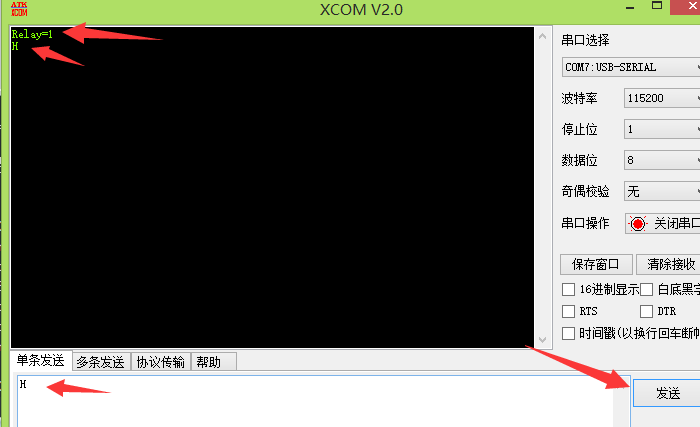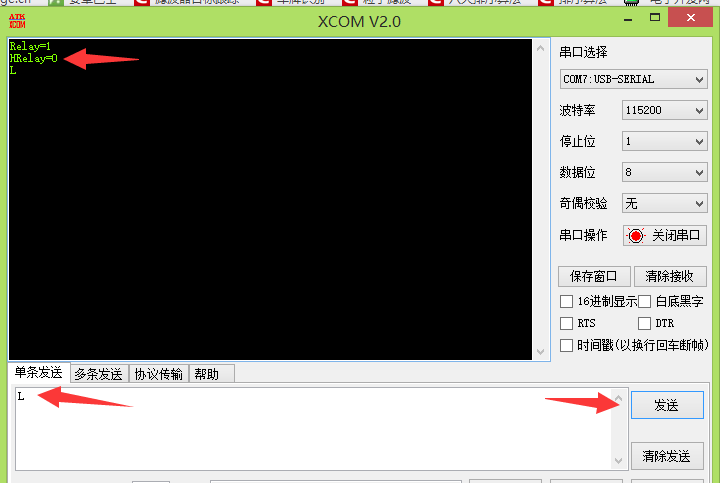[html]
1. gpio.mode(4,gpio.OUTPUT)
2. gpio.write(4,1)
3.
4.
5. tmr.alarm(1, 2000, 0, function()
6.
7.     uart.on("data", 0,function(Revdata)
8.
9.             if Revdata == "++H" then
10.                gpio.write(4,1)
11.                print("Relay=1")
12.             end
13.
14.             if Revdata == "++L" then
15.                gpio.write(4,0)
16.                print("Relay=0")
17.             end
18.
19.             uart.write(0,Revdata) -- unregister callback function
20.
21.     end, 0)
22.
23. end)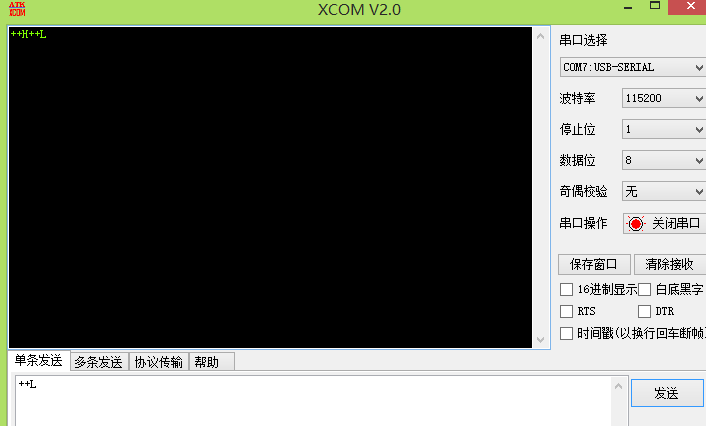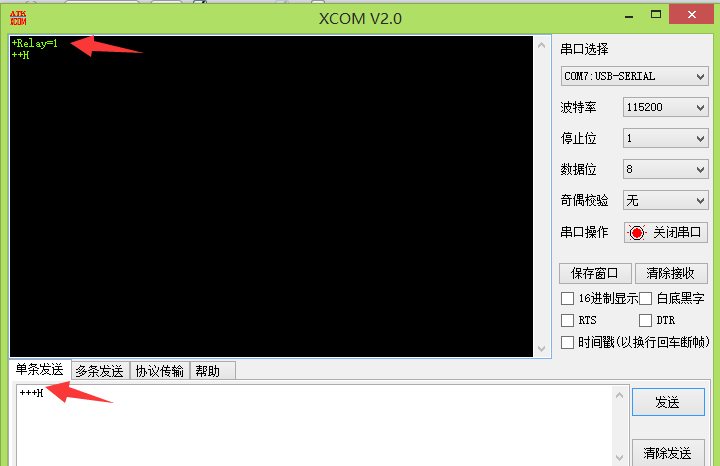[html]
1. gpio.mode(4,gpio.OUTPUT)
2. gpio.write(4,1)
3.
4.
5. tmr.alarm(1, 2000, 0, function()
6.
7.     uart.on("data", 0,function(Revdata)
8.
9.             if Revdata == "++H" then
10.                gpio.write(4,1)
11.                print("Relay=1")
12.             else
13.                print(Revdata)----LOOK  LOOK  LOOK
14.             end
15.
16.             if Revdata == "++L" then
17.                gpio.write(4,0)
18.                print("Relay=0")
19.             end
20.
21.             uart.write(0,Revdata) -- unregister callback function
22.
23.     end, 0)
24.
25. end)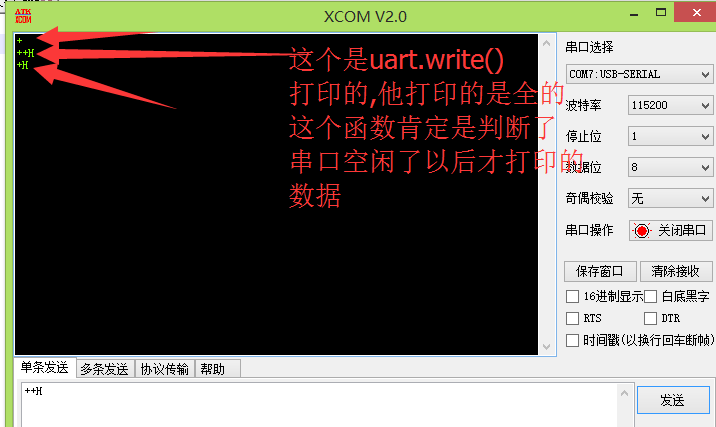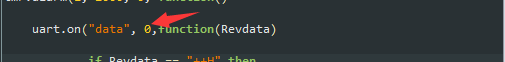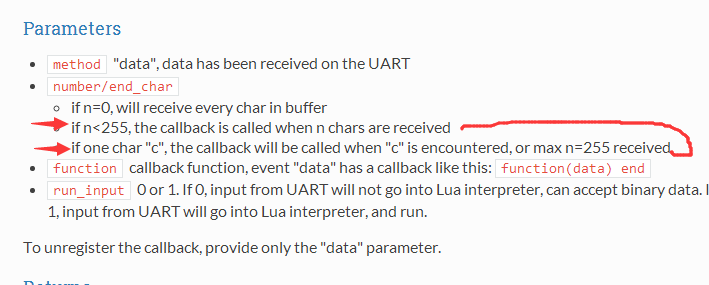[html]
1. gpio.mode(4,gpio.OUTPUT)
2. gpio.write(4,1)
3.
4.
9.
10. tmr.alarm(2, 5, 1, function()
11.
12.     if  ReadCnt ~= 0 then
13.
19.
20.             if  ReadDataCopy == "++H" then
21.                 gpio.write(4,1)
22.             end
23.
24.             if  ReadDataCopy == "++L" then
25.                 gpio.write(4,0)
26.             end
27.
28.         else
30.         end
31.
32.     end
33.
34. end)
35.
36.
37.
38. tmr.alarm(1, 2000, 0, function()
39.
40.     uart.on("data", 0,function(Revdata)
41.
44.     end, 0)
45.
46. end)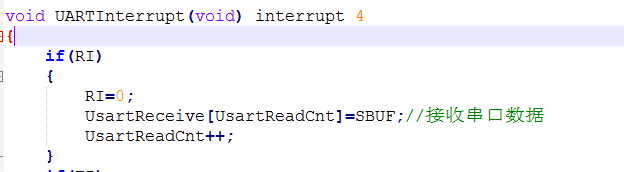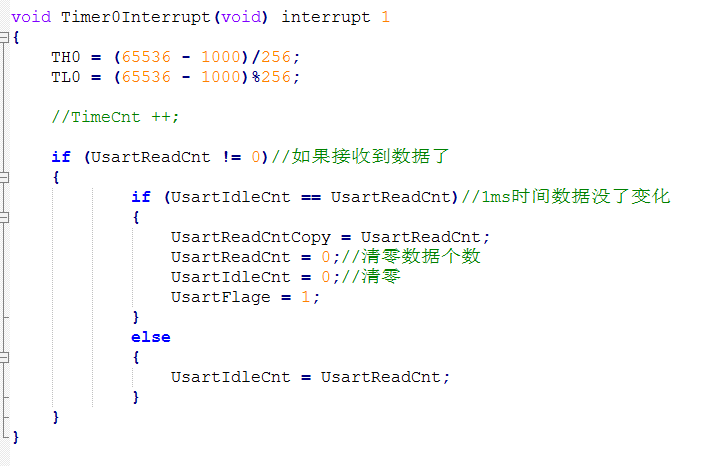https://www.cnblogs.com/yangfengwu/p/7524297.html

posted on 2017-09-14 13:55  杨奉武  阅读(...)  评论(...编辑  收藏